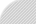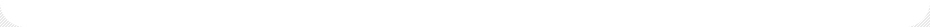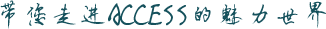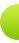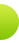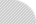Access软件网QQ交流学习群(群号码198465573),欢迎您的加入！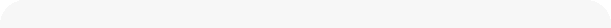优化SQL的执行速度 发表时间：2018/9/5 6:50:52　评论(1)　浏览(1408)　　评论 | 加入收藏 | 复制 摘　要:下面是一些SQL的性能调试方法.整理于网络内容。 正　文:

1. IN和EXISTS

Select name
FROM Personnel
Where birthday IN (Select birthday

FROM Celebrities);

Select P.name
FROM Personnel AS P
Where EXISTS (Select
FROM Clelebrities AS C
Where P.birthday = C.birthday);

COUNT(列名)较快

3. GROUP BY 使用index

GROUP BY col1 如果不能使用index。GROUP BY col1，col2能够使用index的话，改为 GROUP BY col1，col2。

6. 下面的一些写法也会造成使用了index

/* １．index的col_1列有运算 */
Select *
FROM SomeTable
Where col_1 * 1.1 > 100;

Where col_1 IS NULL;

Where SUBSTR(col_1, 1, 1) = 'a';
index的列使用了函数。这个时候可以做个函数index来解决。

Where col_1 <> 100;

Where col_1 > 100 or col_2 = 'abc';
OR的时候最好改为in。 如果非要使用OR的话，追加bitmap index。

× Select * FROM SomeTable Where col_1 LIKE '%a';
× Select * FROM SomeTable Where col_1 LIKE '%a%';
○ Select * FROM SomeTable Where col_1 LIKE 'a%';

Like的时候，只有前方一致能够使用index。

Select * FROM SomeTable Where col_1 = 10;
Select * FROM SomeTable Where col_1 = '10';

Select * FROM SomeTable Where col_1 = CAST(10, AS CHAR(2));

col_1为char类型，类型不匹配的时候，不能使用index。改为类型一致。
○ Select * FROM SomeTable Where col_1 = 10 AND col_2 = 100 AND col_3 = 500;
○ Select * FROM SomeTable Where col_1 = 10 AND col_2 = 100 ;
× Select * FROM SomeTable Where col_1 = 10 AND col_3 = 500 ;
× Select * FROM SomeTable Where col_2 = 100 AND col_3 = 500 ;
× Select * FROM SomeTable Where col_2 = 100 AND col_1 = 10 ;

rowid（Oracle）、oid（PostgreSQL）如果知道行号的话，行号访问最快。

× Select * FROM SomeTable;
○ Select col_1, col_2, col_3 FROM SomeTable;

Access软件网交流QQ群(群号：198465573)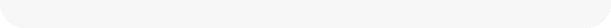相关文章史上速度最快的列表框全选/取消全选方法，10万行1秒内！  【红尘如烟  2010/8/4】Sql优化查询速度50个方法小结\SQL Server速度查询优化...  【风行  2012/8/4】处理上百万条的数据库如何提高处理查询速度（转）  【杜威  2013/12/1】通过视图解决当后台为SQL，客户端使用左右联接导致查询速度很慢。  【易勋  2015/7/2】处理千万级以上的数据提高查询速度的方法  【易勋  2015/7/16】

访客评论2018/9/5陈宏凯： 学习了

发表评论评论内容 (必填) 名字(必填)： 密码(必填)：
 常见问答
 技术分类
 相关资源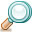文章搜索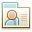关于作者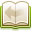文章分类文章存档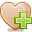友情链接